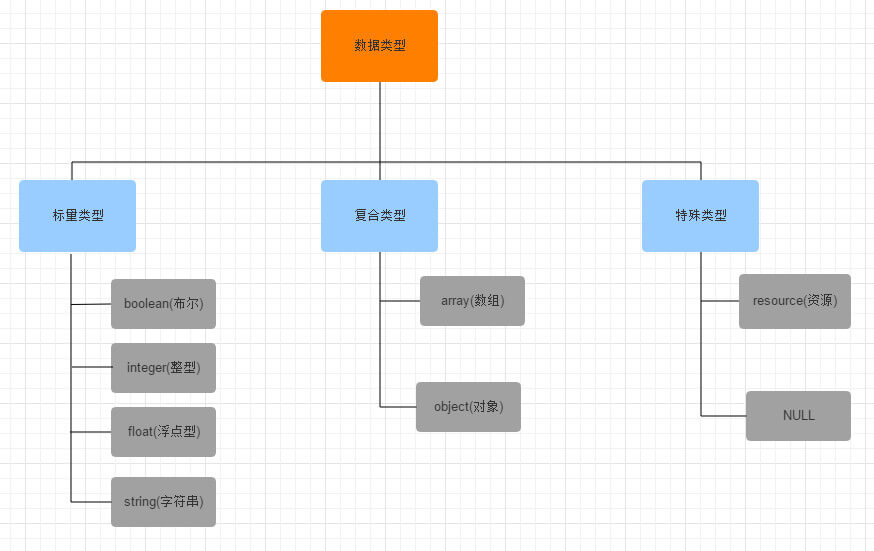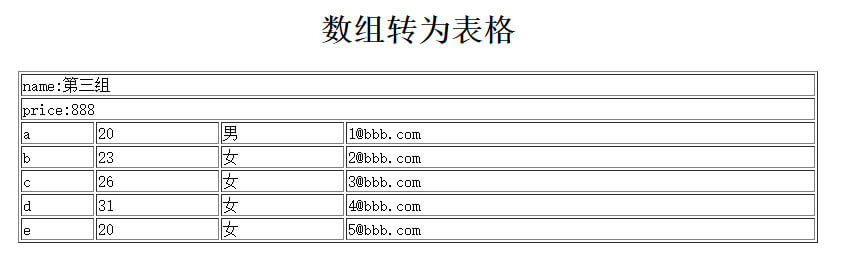# php数据类型

同javascript一样，php也是一门弱类型语言，或者说成类型松散的语言。在强类型语言中，变量要先指定类型，然后才可以对应指定类型的值。而php则不必告知变量的数据类型，会根据它的值，自动把变量转换成正确的数据类型。下面将详细介绍php的数据类型

### 总括

PHP数据类型包括8种：其中包括四种标量类型、两种复合类型和两种特殊类型。具体是：字符串、整数、浮点数、布尔、数组、对象、NULL、资源如果想查看某个表达式的值和类型，可以使用函数var_dump()

```<?php
\$var = 1;
//int 1
echo var_dump(\$var);
echo '<br>';
\$var = 1.0;
//float 1
echo var_dump(\$var);
echo '<br>';
\$var = true;
//boolean true
echo var_dump(\$var);
echo '<br>';
\$var = '1';
//string '1' (length=1)
echo var_dump(\$var);
echo '<br>';
?>```

### 布尔型

布尔型是最简单的类型。boolean表达了真值，可以为TRUE或FALSE

要指定一个布尔值，使用关键字 TRUE 或 FALSE。两个都不区分大小写

```<?php
\$foo = True; // assign the value TRUE to \$foo
?>```

要明确地将一个值转换成 boolean，用 (bool) 或者 (boolean) 来强制转换

当转换为boolean时，以下值被认为是 FALSE：

布尔值 FALSE 本身

整型值 0

浮点型值 0.0

空字符串，以及字符串 "0"

不包括任何元素的数组

不包括任何成员变量的对象(仅 PHP 4.0 适用)

特殊类型 NULL(包括尚未赋值的变量)

从空标记生成的SimpleXML对象

```<?php
var_dump((bool) "");        // bool(false)
var_dump((bool) 1);         // bool(true)
var_dump((bool) -2);        // bool(true)
var_dump((bool) "foo");     // bool(true)
var_dump((bool) 2.3e5);     // bool(true)
var_dump((bool) array(12)); // bool(true)
var_dump((bool) array());   // bool(false)
var_dump((bool) "false");   // bool(true)
?>```

is_bool()函数用来检测变量是否是布尔型

`bool is_bool ( mixed \$var )`

如果 var 是 boolean 则返回 TRUE

```<?php
\$a = false;
\$b = 0;

// 因为 \$a 是布尔型，所以结果为真
if (is_bool(\$a)) {
print "Yes, this is a boolean";
}
// 因为 \$b 不是布尔型，所以结果为非真
if (is_bool(\$b)) {
print "Yes, this is a boolean";
}
?>```

### 整型

PHP整数必须至少有一个数字，不能包含逗号或空格，不能有小数点，正负均可

整型值可以使用十进制，十六进制，八进制或二进制表示，前面可以加上可选的符号(- 或者 +)

二进制表达的 integer 自 PHP 5.4.0 起可用

要使用八进制表达，数字前必须加上 0（零）。要使用十六进制表达，数字前必须加上 0x。要使用二进制表达，数字前必须加上 0b

```<?php
\$a = 1234; // 十进制数
\$a = -123; // 负数
\$a = 0123; // 八进制数(等于十进制 83)
\$a = 0x1A; // 十六进制数(等于十进制 26)
?>```

整型数的字长和平台有关，尽管通常最大值是大约二十亿(32 位有符号)。64位平台下的最大值通常是大约 9E18。PHP不支持无符号整数。Integer 值的字长可以用常量 PHP_INT_SIZE来表示，自 PHP 4.4.0 和 PHP 5.0.5后，最大值可以用常量 PHP_INT_MAX 来表示

```<?php
echo PHP_INT_SIZE;//4
echo PHP_INT_MAX;//2147483647
?>```

如果给定的一个数超出了 integer 的范围，将会被解释为 float。同样如果执行的运算结果超出了 integer 范围，也会返回 float

```<?php
\$large_number = 2147483647;
var_dump(\$large_number);  // int(2147483647)
\$large_number = 2147483648;
var_dump(\$large_number); // float(2147483648)
\$million = 1000000;
\$large_number =  50000 * \$million;
var_dump(\$large_number); // float(50000000000)
?>```

要明确地将一个值转换为 integer，用 (int) 或 (integer) 强制转换。还可以通过函数 intval() 来将一个值转换成整型

```<?php
echo intval(42);                      // 42
echo intval(4.2);                     // 4
echo intval('+42');                   // 42
echo intval('-42');                   // -42
echo intval(042);                     // 34
echo intval('042');                   // 42
echo intval(1e10);                    // 1410065408
echo intval('1e10');                  // 1
echo intval(0x1A);                    // 26
echo intval(420000000000000000000);   // 0
echo intval(42, 8);                   // 42
echo intval('42', 8);                 // 34
echo intval(array());                 // 0
echo intval(array('foo', 'bar'));     // 1
?>```

从布尔值转换，FALSE 将产生出 0，TRUE 将产生出 1；从浮点型转换，当从浮点数转换成整数时，将向下取整；从字符串转换，如果该字符串没有包含 '.'，'e' 或 'E' 并且其数字值在整型的范围之内，该字符串将被当成 integer 来取值。其它所有情况下都被作为float来取值。该字符串的开始部分决定了它的值。如果该字符串以合法的数值开始，则使用该数值。否则其值为0。合法数值由可选的正负号，后面跟着一个或多个数字(可能有小数点)，再跟着可选的指数部分。指数部分由'e'或'E'后面跟着一个或多个数字构成

```<?php
echo (int)true;//1
echo (int)false;//0
echo (int)1.6;//1
echo (int)'1.6px';//1
echo (int)'px';//0
?>```

is_int()、is_integer()、is_long()这三个函数可以用来检测变量是否是整数

```<?php
\$a = 1;
\$b = 1.0;

var_dump(is_int(\$a));//true
var_dump(is_int(\$b));//false
var_dump(is_integer(\$a));//true
var_dump(is_integer(\$b));//false
var_dump(is_long(\$a));//true
var_dump(is_long(\$b));//false
?>```

### 浮点型

浮点型（也叫浮点数 float，双精度数 double 或实数 real）

```<?php
\$a = 1.234;
\$b = 1.2e3;
\$c = 7E-10;
?>```

PHP 通常使用 IEEE 754 双精度格式，则由于取整而导致的最大相对误差为 1.11e-16。非基本数学运算可能会给出更大误差，并且要考虑到进行复合运算时的误差传递

以十进制能够精确表示的有理数如 0.1 或 0.7，无论有多少尾数都不能被内部所使用的二进制精确表示，因此不能在不丢失一点点精度的情况下转换为二进制的格式。这就会造成混乱的结果：例如，floor((0.1+0.7)*10) 通常会返回 7 而不是预期中的 8，因为该结果内部的表示其实是类似 7.9999999999999991118...

```<?php
echo floor((0.1+0.7)*10);//7
?>```

要明确地将一个值转换为 float，用(float)强制转换。还可以通过函数 floatval()、doubleval()来将一个值转换成整型。除了字符串类型之外，一般地，都是先将值转换成整型，然后再转换成浮点数

```<?php
\$var1 = '122.34343The';
\$float_value1_of_var1 = floatval (\$var1);
\$float_value1_of_var1 = doubleval (\$var1);
print \$float_value1_of_var1; // 打印出 122.34343
print \$float_value1_of_var1; // 打印出 122.34343

\$var2 = true;
\$float_value2_of_var2 = floatval (\$var2);
\$float_value2_of_var2 = doubleval (\$var2);
print \$float_value2_of_var2; // 打印出 1
print \$float_value2_of_var2; // 打印出 1
?>```

比较两个浮点数是否相等是有问题的。不过还是有迂回的方法来比较浮点数值的

要测试浮点数是否相等，要使用一个仅比该数值大一丁点的最小误差值。该值也被称为机器极小值（epsilon）或最小单元取整数，是计算中所能接受的最小的差别值

```<?php
\$a = 1.23456789;
\$b = 1.23456780;
\$epsilon = 0.00001;

if(abs(\$a-\$b) < \$epsilon) {
echo "true";
}
?>```

NaN

某些数学运算会产生一个由常量 NAN 所代表的结果。此结果代表着一个在浮点数运算中未定义或不可表述的值。任何拿此值与其它任何值进行的松散或严格比较的结果都是 FALSE

由于 NAN 代表着任何不同值，不应拿 NAN 去和其它值进行比较，包括其自身，应该用 is_nan() 来检查

```<?php
\$nan = acos(8);
var_dump(\$nan, is_nan(\$nan));//float NAN boolean true
?>```

is_real()、is_float()这两个函数可以用来检测变量是否是浮点数

```<?php
isfloat("5.0" + 0);  // true
isfloat("5.0");  // false
isfloat(5 + 0);  // false
isfloat(5.0 + 0);  // false
isfloat('a' + 0);  // false
?>```

### 字符串

一个字符串string就是由一系列的字符组成，其中每个字符等同于一个字节

一个字符串可以用 4 种方式表达：单引号、双引号、heredoc语法结构、nowdoc语法结构(自 PHP 5.3.0 起)

定义一个字符串的最简单的方法是用单引号把它包围起来

要表达一个单引号自身，需在它的前面加个反斜线()来转义。要表达一个反斜线自身，则用两个反斜线(\)。其它任何方式的反斜线都不会被当成反斜线：也就是说如果想使用其它转义序列例如 \r 或者 \n，并不代表任何特殊含义，就单纯是这两个字符本身

[注意]在单引号字符串中的变量和特殊字符的转义序列将不会被替换

```<?php
echo 'this is a simple string';
// 可以录入多行
echo 'You can also have embedded newlines in
strings this way as it is
okay to do';
// 输出： Arnold once said: "I'll be back"
echo 'Arnold once said: "I\'ll be back"';
// 输出： You deleted C:\*.*?
echo 'You deleted C:\\*.*?';
// 输出： You deleted C:\*.*?
echo 'You deleted C:\*.*?';
// 输出： This will not expand: \n a newline
echo 'This will not expand: \n a newline';
// 输出： Variables do not \$expand \$either
echo 'Variables do not \$expand \$either';
?>```

如果字符串是包围在双引号中， PHP将对一些特殊的字符进行解析。用双引号定义的字符串最重要的特征是变量会被解析

【转义字符】

```序列       含义
\n        换行(ASCII 字符集中的 LF 或 0x0A (10))
\r        回车(ASCII 字符集中的 CR 或 0x0D (13))
\t        水平制表符(ASCII 字符集中的 HT 或 0x09 (9))
\v        垂直制表符(ASCII 字符集中的 VT 或 0x0B (11))(自 PHP 5.2.5 起)
\e        Escape(ASCII 字符集中的 ESC 或 0x1B (27))(自 PHP 5.4.0 起)
\f        换页(ASCII 字符集中的 FF 或 0x0C (12))(自 PHP 5.2.5 起)
\\        反斜线
\\$        美元标记
\"        双引号
\[0-7]{1,3}    符合该正则表达式序列的是一个以八进制方式来表达的字符
\x[0-9A-Fa-f]{1,2}    符合该正则表达式序列的是一个以十六进制方式来表达的字符```

Heredoc结构

第三种表达字符串的方法是用heredoc句法结构(又叫做定界符)：<<<。在该运算符之后要提供一个标识符，然后换行。接下来是字符串string 本身，最后要用前面定义的标识符作为结束标志

结束时所引用的标识符必须在该行的第一列，而且，标识符的命名也要像其它标签一样遵守 PHP 的规则：只能包含字母、数字和下划线，并且必须以字母和下划线作为开头

[注意]结束标识符这行除了可能有一个分号外，绝对不能包含其它字符。这意味着标识符不能缩进，分号的前后也不能有任何空白或制表符

```<?php
\$str = <<< G
123
G;
echo \$str;//123
?>```

Heredoc结构就像是没有使用双引号的双引号字符串，这就是说在heredoc 结构中单引号不用被转义，但是转义序列还可以使用。变量将被替换

```<?php
\$str = <<<EOD
Example of string
spanning multiple lines
using heredoc syntax.
EOD;
class foo
{
var \$foo;
var \$bar;
function foo()
{
\$this->foo = 'Foo';
\$this->bar = array('Bar1', 'Bar2', 'Bar3');
}
}
\$foo = new foo();
\$name = 'MyName';
/*My name is "MyName". I am printing some Foo.
Now, I am printing some Bar2.
This should print a capital 'A': A*/
echo <<<EOT
My name is "\$name". I am printing some \$foo->foo.
Now, I am printing some {\$foo->bar}.
This should print a capital 'A': \x41
EOT;
?>```

Nowdoc结构

就像 heredoc 结构类似于双引号字符串，Nowdoc 结构是类似于单引号字符串的。Nowdoc 结构很像 heredoc 结构，但是 nowdoc 中不进行解析操作。这种结构很适合用于嵌入 PHP 代码或其它大段文本而无需对其中的特殊字符进行转义

```<?php
\$str = <<<'EOD'
Example of string
spanning multiple lines
using nowdoc syntax.
EOD;
class foo
{
public \$foo;
public \$bar;
function foo()
{
\$this->foo = 'Foo';
\$this->bar = array('Bar1', 'Bar2', 'Bar3');
}
}
\$foo = new foo();
\$name = 'MyName';
/*
My name is "\$name". I am printing some \$foo->foo.
Now, I am printing some {\$foo->bar}.
This should not print a capital 'A': \x41
*/
echo <<<'EOT'
My name is "\$name". I am printing some \$foo->foo.
Now, I am printing some {\$foo->bar}.
This should not print a capital 'A': \x41
EOT;
?>```

一个值可以通过在其前面加上 (string) 或用 strval() 函数来转变成字符串。在一个需要字符串的表达式中，会自动转换为string。比如在使用函数echo或print时，或在一个变量和一个 string 进行比较时，就会发生这种转换

NULL 总是被转变成空字符串

一个布尔值 boolean 的 TRUE 被转换成 string 的 "1"。Boolean 的 FALSE 被转换成 ""（空字符串）。这种转换可以在 boolean 和 string 之间相互进行

一个整数 integer 或浮点数 float 被转换为数字的字面样式的 string（包括 float 中的指数部分）。使用指数计数法的浮点数（4.1E+6）也可转换

当一个字符串被当作一个数值来取值，其结果和类型如下

如果该字符串没有包含 '.'，'e' 或 'E' 并且其数字值在整型的范围之内（由 PHP_INT_MAX 所定义），该字符串将被当成 integer 来取值。其它所有情况下都被作为 float 来取值

该字符串的开始部分决定了它的值。如果该字符串以合法的数值开始，则使用该数值。否则其值为 0（零）。合法数值由可选的正负号，后面跟着一个或多个数字（可能有小数点），再跟着可选的指数部分。指数部分由 'e' 或 'E' 后面跟着一个或多个数字构成

```<?php
\$foo = 1 + "10.5";                // \$foo is float (11.5)
\$foo = 1 + "-1.3e3";              // \$foo is float (-1299)
\$foo = 1 + "bob-1.3e3";           // \$foo is integer (1)
\$foo = 1 + "bob3";                // \$foo is integer (1)
\$foo = 1 + "10 Small Pigs";       // \$foo is integer (11)
\$foo = 4 + "10.2 Little Piggies"; // \$foo is float (14.2)
\$foo = "10.0 pigs " + 1;          // \$foo is float (11)
\$foo = "10.0 pigs " + 1.0;        // \$foo is float (11)
?>```

直接把 array，object 或 resource 转换成 string 不会得到除了其类型之外的任何有用信息

is_string()函数用来检测变量是否是字符串

`bool is_string ( mixed \$var )`

如果 var 是 string 则返回 TRUE，否则返回 FALSE

```<?php
var_dump(is_string('123'));//boolean true
var_dump(is_string(123));//boolean false
?>```

### 数组

PHP 中的数组实际上是一个有序映射。映射是一种把 values 关联到 keys 的类型

由于数组元素的值也可以是另一个数组，树形结构和多维数组也是允许的

可以用 array() 语言结构来新建一个数组。它接受任意数量用逗号分隔的 键（key） => 值（value）对

```array(  key =>  value
, ...
)
// 键（key）可是是一个整数 integer 或字符串 string
// 值（value）可以是任意类型的值```
```<?php
\$array = array(
"foo" => "bar",
"bar" => "foo",
);

// 自 PHP 5.4 起
\$array = [
"foo" => "bar",
"bar" => "foo",
];
?>```

key可以是integer或者string。value可以是任意类型

此外key会有如下的强制转换

包含有合法整型值的字符串会被转换为整型。例如键名 "8" 实际会被储存为 8。但是 "08" 则不会强制转换，因为其不是一个合法的十进制数值

浮点数也会被转换为整型，意味着其小数部分会被舍去。例如键名 8.7 实际会被储存为 8

布尔值也会被转换成整型。即键名 true 实际会被储存为 1 而键名 false 会被储存为 0

Null 会被转换为空字符串，即键名 null 实际会被储存为 ""

数组和对象不能被用为键名

如果在数组定义中多个单元都使用了同一个键名，则只使用了最后一个，之前的都被覆盖了

```<?php
\$array = array(
1    => "a",
"1"  => "b",
1.5  => "c",
true => "d",
);
/*
array(1) {
=>
string(1) "d"
}
*/
var_dump(\$array);
?>```

key 为可选项。如果未指定，PHP 将自动使用之前用过的最大 integer 键名加上 1 作为新的键名

```<?php
\$array = array("foo", "bar", "hallo", "world");
/*
array(4) {
=>
string(3) "foo"
=>
string(3) "bar"
=>
string(5) "hallo"
=>
string(5) "world"
}
*/
var_dump(\$array);
?>

<?php
\$array = array(
"a",
"b",
6 => "c",
"d",
);
/*
array(4) {
=>
string(1) "a"
=>
string(1) "b"
=>
string(1) "c"
=>
string(1) "d"
}
*/
var_dump(\$array);
?>```

数组单元可以通过 array[key] 语法来访问

[注意]数组的中括号[]可以用花括号{}代替

```<?php
\$array = array(
"foo" => "bar",
42    => 24,
"multi" => array(
"dimensional" => array(
"array" => "foo"
)
)
);
/*
string(3) "bar"
int(24)
string(3) "foo"
*/
var_dump(\$array["foo"]);
var_dump(\$array);
var_dump(\$array["multi"]["dimensional"]["array"]);
?>```

要修改某个值，通过其键名给该单元赋一个新值。要删除某键值对，对其调用 unset() 函数

[注意]如果给出方括号但没有指定键名，则取当前最大整数索引值，新的键名将是该值加上 1（但是最小为 0）。如果当前还没有整数索引，则键名将为 0

```<?php
\$arr = array(5 => 1, 12 => 2);
\$arr[] = 56;    // This is the same as \$arr = 56;
// at this point of the script
\$arr["x"] = 42; // This adds a new element to
// the array with key "x"
unset(\$arr); // This removes the element from the array
unset(\$arr);    // This deletes the whole array
?>```

如果将数组中的某个值置为null，则value为空，键值仍然存在

```<?php
\$arr = [0,1,2,3];
\$arr = null;
/*
array (size=4)
0 => int 0
1 => null
2 => int 2
3 => int 3
*/
var_dump(\$arr);
?>```

如果将数组中的某个值使用unset()，则键值对都不存在

```<?php
\$arr = [0,1,2,3];
unset(\$arr);
/*
array (size=3)
0 => int 0
2 => int 2
3 => int 3
*/
var_dump(\$arr);
?>```

【array_values】

array_values() 函数可以返回数组中所有的值并给其建立数字索引

```<?php
\$arr = [0,1,2,3];
unset(\$arr);
\$arr = array_values(\$arr);
/*
array (size=3)
0 => int 0
1 => int 2
2 => int 3
*/
var_dump(\$arr);
?>```

foreach 语法结构提供了遍历数组的简单方式。foreach 仅能够应用于数组和对象，如果尝试应用于其他数据类型的变量，或者未初始化的变量将发出错误信息

```<?php
\$arr = array(1, 2, 3, 4);
foreach (\$arr as &\$value) {
\$value = \$value * 2;
}
// \$arr is now array(2, 4, 6, 8)
unset(\$value); // 最后取消掉引用
?>```

一般地，可以使用foreach()语句将二维数组遍历成一个表格的形式

```<?php
\$group = [
"name"=>"第三组",
"price"=>888,
["name"=>"a", "age"=>20, "sex"=>"男", "email"=>"1@bbb.com"],
["name"=>"b", "age"=>23, "sex"=>"女", "email"=>"2@bbb.com"],
["name"=>"c", "age"=>26, "sex"=>"女", "email"=>"3@bbb.com"],
["name"=>"d", "age"=>31, "sex"=>"女", "email"=>"4@bbb.com"],
["name"=>"e", "age"=>20, "sex"=>"女", "email"=>"5@bbb.com"]
];

echo '<table border="1" width="800" align="center">';
echo '<caption><h1>数组转为表格<h1></caption>';
foreach(\$group as \$k=>\$row) {
echo '<tr>';
if(is_array(\$row)) {
foreach(\$row as \$col) {
echo '<td>'.\$col.'</td>';
}
} else {
echo '<td colspan="4">'.\$k.':'.\$row.'</td>';
}
echo '</tr>';
}
echo '</table>';
?>```【list()】

list()函数可以将数组中的值赋给变量

```list(\$a,\$b,\$c) = [1,2,3];
echo \$a;//1
echo \$b;//2
echo \$c;//3
// 仅接收其中一个
list(, , \$power) = [1,2,3];
echo \$power;//3
// list() 不能对字符串起作用
list(\$bar) = "abcde";
var_dump(\$bar); // NULL```

【each()】

each()函数用于返回数组中当前的键／值对并将数组指针向前移动一步

[注意]如果内部指针越过了数组的末端，则 each() 返回 FALSE

```<?php
\$foo = array("bob", "fred", "jussi", "jouni");
\$bar = each(\$foo);
//Array (  => bob [value] => bob  => 0 [key] => 0 )
print_r(\$bar);
?>```

each()函数常常和list()配合来遍历数组

```<?php
\$foo = array("bob", "fred", "jussi", "jouni");
while(list(\$key,\$val) = each(\$foo)){
//0 => bob 1 => fred 2 => jussi 3 => jouni
echo "\$key => \$val\n";
}
?>```

在执行each() 之后，数组指针将停留在数组中的下一个单元或者当碰到数组结尾时停留在最后一个单元。如果要再用each 遍历数组，必须使用 reset()

```<?php
\$foo = array("bob", "fred", "jussi", "jouni");
while(list(\$key,\$val) = each(\$foo)){
//0 => bob 1 => fred 2 => jussi 3 => jouni
echo "\$key => \$val\n";
}
reset(\$foo);
while(list(\$key,\$val) = each(\$foo)){
//0 => bob 1 => fred 2 => jussi 3 => jouni
echo "\$key => \$val\n";
}
?>```

前面提到的reset()函数就是数组内部指针函数的一种，数组内部指针函数包括key()、current()、next()、prev()、end()和reset()

```key()      获取当前下标
current()  获取当前值
next()     指针向后移动
prev()     指针向左移动
reset()    指针指向数组中第一个元素
end()      指针指向数组中最后一个元素```
```<?php
\$arr = ['a','b','c','d'];
echo key(\$arr);//0
echo current(\$arr);//a
echo next(\$arr);//b
echo key(\$arr);//1
echo current(\$arr);//b
echo end(\$arr);//d
echo key(\$arr);//3
echo current(\$arr);//d
echo prev(\$arr);//c
echo key(\$arr);//2
echo current(\$arr);//c
echo reset(\$arr);//a
echo key(\$arr);//0
echo current(\$arr);//a
?>```

将 NULL 转换为 array 会得到一个空的数组

对于任意 integer，float，string，boolean 和 resource 类型，如果将一个值转换为数组，将得到一个仅有一个元素的数组，其下标为 0，该元素即为此标量的值

如果一个 object 类型转换为 array，则结果为一个数组，其单元为该对象的属性。键名将为成员变量名，不过有几点例外：整数属性不可访问；私有变量前会加上类名作前缀；保护变量前会加上一个 '*' 做前缀。这些前缀的前后都各有一个 NULL 字符

array_diff()函数用来计算数组的差集

`array array_diff ( array \$array1 , array \$array2 [, array \$... ] )`

对比返回在 array1 中但是不在 array2 及任何其它参数数组中的值

```<?php
\$array1 = array("a" => "green", "red", "blue", "red");
\$array2 = array("b" => "green", "yellow", "red");
\$result = array_diff(\$array1, \$array2);
/*
Array
(
 => blue
)
*/
print_r(\$result);
?>```

is_array()函数用来检测变量是否是数组

```<?php
var_dump(is_array([]));//boolean true
var_dump(is_array(''));//boolean false
?>```

### 对象

要创建一个新的对象 object，使用 new 语句实例化一个类

```<?php
class foo
{
function do_foo()
{
echo "Doing foo.";
}
}
\$bar = new foo;
\$bar->do_foo();
?>```

如果将一个对象转换成对象，它将不会有任何变化。如果其它任何类型的值被转换成对象，将会创建一个内置类 stdClass 的实例。如果该值为 NULL，则新的实例为空。数组转换成对象将使键名成为属性名并具有相对应的值。对于任何其它的值，名为 scalar 的成员变量将包含该值

```<?php
\$obj = (object) 'ciao';
echo \$obj->scalar;  // outputs 'ciao'
?>```

is_object()方法用来检测变量是否是一个对象

```<?php
var_dump(is_object(''));//boolean false
var_dump(is_object((object)''));//boolean true
?>```

### NULL

PHP中的NULL是空类型，对大小写不敏感，NULL类型只有一个取值，表示一个变量没有值，当被赋值为NULL，或者尚未被赋值，或者被unset()，这三种情况下变量被认为为NULL

```<?php
error_reporting(0); //禁止显示PHP警告提示
\$var;
var_dump(\$var);//NULL
\$var1 = null;
var_dump(\$var1);//NULL
\$var2 = NULL;
var_dump( \$var2);//NULL
\$var3 = "节日快乐！";
unset(\$var3);
var_dump(\$var3);//NULL
?>```

使用 (unset) \$var 将一个变量转换为 null 将不会删除该变量或 unset 其值。仅是返回 NULL 值而已

is_null()函数用来检测变量是否为 NULL

```<?php
var_dump(is_null(NULL));//boolean true
var_dump(is_null(''));//boolean false
?>```

### 资源

PHP资源是由专门的函数来建立和使用的，例如打开文件、数据连接、图形画布。可以对资源进行操作（创建、使用和释放）。任何资源，在不需要的时候应该被及时释放。如果我们忘记了释放资源，系统自动启用垃圾回收机制，在页面执行完毕后回收资源，以避免内存被消耗殆尽

```<?php
\$file=fopen("data/webroot/resource/f.txt","r");   //打开文件
\$con=mysql_connect("127.0.0.1","root","root");  //连接数据库
if (\$file_handle){
//接着采用while循环（后面语言结构语句中的循环结构会详细介绍）一行行地读取文件，然后输出每行的文字
while (!feof(\$file_handle)) { //判断是否到最后一行
\$line = fgets(\$file_handle); //读取一行文本
echo \$line; //输出一行文本
echo "<br />"; //换行
}
}
fclose(\$file_handle);//关闭文件
?>```